# Calculating Yield on Debt

How can we evaluate the performance of debt investments?

## How can we calculate Yield on Debt?

Debt yield refers to the rate of return an investor can expect to earn if he/she holds a debt instrument until maturity. Such instruments include government-backed T-bills, corporate bonds, private debt agreements, and other fixed income securities. In this article, we will explore the four different types of yields: Bank Discount, Holding Period, Money Market, and Effective Annual.### Bank Discount Yield (BDY)

The BDY formula is best suited to calculating yield on short-term debt instruments such as government T-bills. The formula for calculating BDY is: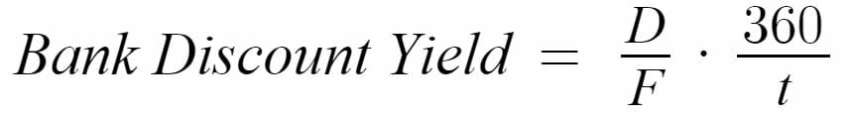Where:

D – Discount/premium from face value (face value – market price)

F – Face value

360 – Number of days in a year (as per banking conventions)

– Number of days until maturity

#### BDY Example

An investor wants to buy a U.S. T-bill. The current market price is \$490 and the security has a face value of \$500. The T-bill matures in 200 days from today. Should the investor buy the T-bill today, what is the expected BDY?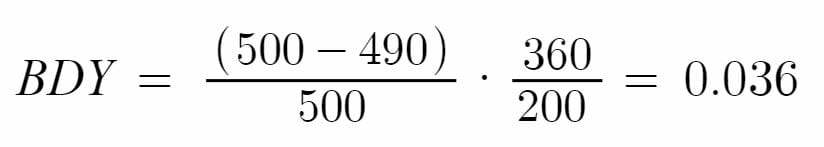The BDY on this investment is 3.6%.

### Holding Period Yield (HPY)

HPY measures any capital gains and/or losses from debt investments that have occurred over a specified holding period. The formula for calculating HPY is: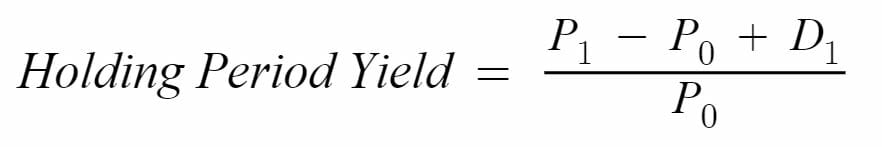Where:

P1 – Price of security at the end of the holding period (maturity)

P0 – Price of security at the beginning of the holding period (purchase price)

D1 – Cash distributions during holding period (coupons)

#### HPY Example

An investor buys a T-bill at a face value for \$500. He then sells the T-bill at maturity for \$510. What is his HPY?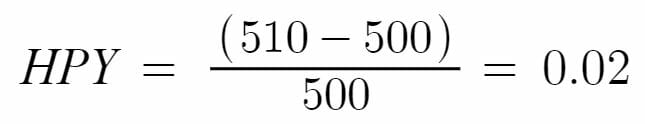The BDY on this investment is 2%. Note that T-bills are zero-coupon securities and do not pay a monthly coupon to investors. Thus, the D1 term in this example is equal to 0.

### Money Market Yield (MMY)

MMY calculates the return on highly liquid, short-term debt instruments such as certificates of deposits, commercial paper, or T-bills. MMY is different from BDY, as it computes yield based on the purchase price of the security rather than the security’s face value. The equation for MMY is: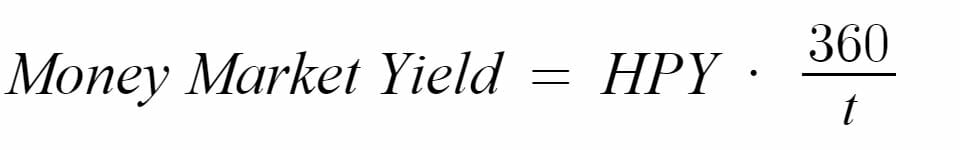Where:

360 – Number of days in a year (as per banking conventions)

– Number of days until maturity

#### MMY Example

This example uses the same numbers as the HPY example above. Now, we learn that the T-bill was a 3-month T-bill that matures in 90 days. What is the investment’s MMY?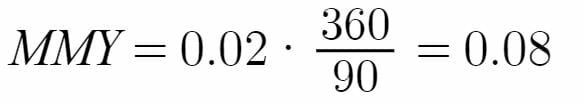Using the HPY that we calculated earlier, the MMY on this investment is 8%.

### Effective Annual Yield (EAY)

EAY is essentially the annualized version of HPY. It provides a number that is easily comparable to the annual returns of other securities. The equation for EAY is: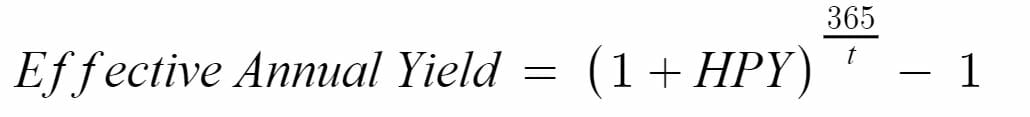Where:

365 – Number of days in the year (different from bank convention)

– Number of days until maturity

#### EAY Example

This example uses the same numbers as the HPY and MMY examples above, where HPY is 0.02 and t is 90 days.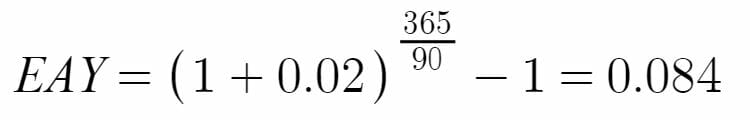The annualized return for this investment is 8.4%.

### More Resources

We hope you enjoyed CFI’s explanation of yield on debt. CFI offers the Financial Modeling & Valuation Analyst (FMVA)™ certification program for those looking to take their careers to the next level. To learn more about related topics, check out the following resources:

• Convertible Bond
• Risk-Free Rate